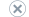# Microsoft Linear Regression Algorithm

Applies to:SQL Server 2019 and earlier Analysis ServicesAzure Analysis ServicesPower BI Premium

Important

Data mining was deprecated in SQL Server 2017 Analysis Services and now discontinued in SQL Server 2022 Analysis Services. Documentation is not updated for deprecated and discontinued features. To learn more, see Analysis Services backward compatibility.

The Microsoft Linear Regression algorithm is a variation of the Microsoft Decision Trees algorithm that helps you calculate a linear relationship between a dependent and independent variable, and then use that relationship for prediction.

The relationship takes the form of an equation for a line that best represents a series of data. For example, the line in the following diagram is the best possible linear representation of the data.Each data point in the diagram has an error associated with its distance from the regression line. The coefficients a and b in the regression equation adjust the angle and location of the regression line. You can obtain the regression equation by adjusting a and b until the sum of the errors that are associated with all the points reaches its minimum.

There are other kinds of regression that use multiple variables, and also nonlinear methods of regression. However, linear regression is a useful and well-known method for modeling a response to a change in some underlying factor.

## Example

You can use linear regression to determine a relationship between two continuous columns. For example, you can use linear regression to compute a trend line from manufacturing or sales data. You could also use the linear regression as a precursor to development of more complex data mining models, to assess the relationships among data columns.

Although there are many ways to compute linear regression that do not require data mining tools, the advantage of using the Microsoft Linear Regression algorithm for this task is that all the possible relationships among the variables are automatically computed and tested. You do not have to select a computation method, such as solving for least squares. However, linear regression might oversimplify the relationships in scenarios where multiple factors affect the outcome.

## How the Algorithm Works

The Microsoft Linear Regression algorithm is a variation of the Microsoft Decision Trees algorithm. When you select the Microsoft Linear Regression algorithm, a special case of the Microsoft Decision Trees algorithm is invoked, with parameters that constrain the behavior of the algorithm and require certain input data types. Moreover, in a linear regression model, the whole data set is used for computing relationships in the initial pass, whereas a standard decision trees model splits the data repeatedly into smaller subsets or trees.

## Data Required for Linear Regression Models

When you prepare data for use in a linear regression model, you should understand the requirements for the particular algorithm. This includes how much data is needed, and how the data is used. The requirements for this model type are as follows:

• A single key column Each model must contain one numeric or text column that uniquely identifies each record. Compound keys are not permitted.

• A predictable column Requires at least one predictable column. You can include multiple predictable attributes in a model, but the predictable attributes must be continuous numeric data types. You cannot use a datetime data type as a predictable attribute even if the native storage for the data is numeric.

• Input columns Input columns must contain continuous numeric data and be assigned the appropriate data type.

For more information, see the Requirements section of Microsoft Linear Regression Algorithm Technical Reference.

## Viewing a Linear Regression Model

To explore the model, you use the Microsoft Tree Viewer. The tree structure for a linear regression model is very simple, with all the information about the regression equation contained in a single node. For more information, see Browse a Model Using the Microsoft Tree Viewer.

If you want to know more detail about the equation, you can also view the coefficients and other details by using the Microsoft Generic Content Tree Viewer.

For a linear regression model, the model content includes metadata, the regression formula, and statistics about the distribution of input values. For more information, see Mining Model Content for Linear Regression Models (Analysis Services - Data Mining).

## Creating Predictions

After the model has been processed, the results are stored as a set of statistics together with the linear regression formula, which you can use to compute future trends. For examples of queries to use with a linear regression model, see Linear Regression Model Query Examples.

For general information about how to create queries against mining models, see Data Mining Queries.

In addition to creating a linear regression model by selecting the Microsoft Linear Regression algorithm, if the predictable attribute is a continuous numeric data type, you can create a decision tree model that contains regressions. In this case, the algorithm will split the data when it finds appropriate separation points, but for some regions of data, will create a regression formula instead. For more information about regression trees within a decision trees model, see Mining Model Content for Decision Tree Models (Analysis Services - Data Mining).

## Remarks

• Does not support the use of Predictive Model Markup Language (PMML) to create mining models.

• Does not support the creation of data mining dimensions.

• Supports drillthrough.

• Supports the use of OLAP mining models.